# Waves

on the surface of a liquid

Deviations of the surface of the liquid from the state of equilibrium which propagate under the action of forces tending to restore this state. Depending on the nature of these forces — surface tension or gravity — waves on the surface of a liquid are divided into capillary and gravitational waves.

The theory of gravitational waves has been best developed for potential motions of the liquid, in particular for plane-parallel motions; the bulk of forces applied to the particles of the liquid are gravitational forces. The determination of the velocity potential of the wave motion involves the integration of the Laplace equation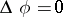under boundary conditions of a special kind. The pressure is constant all over the surface of the liquid, the equation of which,, may be found after the problem has been solved. From the well-known integral of hydrodynamic equations, one then finds the first boundary condition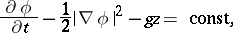(1)

which should be satisfied for. The second boundary condition follows from the fact that the surface of the liquid contains the same particles throughout the period of the motion. Namely, for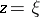: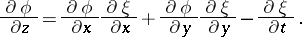(2)

In addition, another requirement which must be met is that the flow is tangential to the solid bodies in the liquid. The following initial conditions must also be satisfied at the same time as the boundary conditions: At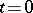the particles of the liquid must have specified initial velocities, while the surface of the liquid must have a given shape. This amounts to stipulating the velocity potential atas a function of,andand specifying the function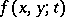at.

The principal difficulty of the problem is that all the above conditions must be satisfied on a surface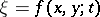whose equation can only be found after the problem itself has been solved. In this respect problems in the theory of waves have much in common with problems in the theory of jets and equilibrium shapes of rotating liquids.

Since a rigorous solution of the problems of wave theory under the above boundary conditions is practically impossible, the theory of infinitely-small waves was developed instead. This theory assumes that the velocities of the particles of the liquid and the deviations of the surface of the liquid from the horizontal equilibrium level are small. Under this assumption, the boundary conditions (1) and (2) assume the form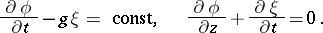It is allowed, in this context, to replace the variablein the partial derivatives of the velocity potential by zero. Under such assumptions, the boundary condition for the functionassumes the form: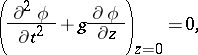while the equation of the wavy surface assumes the form: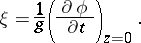The principal results arrived at in the theory of infinitely-small waves made it possible to deal with many important problems of geophysics and to clarify, for example, the laws governing the propagation of tidal waves in the Ocean. The theory of infinitely-small waves yielded the solution of problems of wave formation by sailing vessels and made it possible to produce a hydrodynamic theory of a ship's roll in a swell. Extensive application of methods of the theory of functions of a complex variable made it possible to solve a number of very complicated problems on the propagation of waves in water reservoirs of variable depth, and on diffraction and reflection of waves off floating bodies.

The theory of waves of finite amplitude was developed by A.I. Nekrasov , who found the shape of periodic waves by solving rigorously the planar problem with the boundary conditions (1) and (2). The determination of the respective velocity potential had been reduced to finding a function representing the conformal mapping of the domain occupied by a single wave into a disc or an annulus. This function is found by solving a certain non-linear integral equation.

Owing to the development of the theory of non-linear boundary problems and integral equations, the theory of waves with finite amplitudes was enriched with new results. In particular, it was demonstrated that there exist a solitary wave and a limit Stokes wave with an angular point on its profile. In the theory of standing waves with finite amplitude, i.e. of periodic eigenvibrations of the surface of the liquid, there exists only an approximate solution, based on the use of Lagrange variables.

The determination of standing waves and travelling waves on the surface of a three-dimensional flow is a problem which is difficult to solve even in its approximate form.